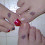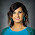## 1.22.2011

### Concept Attainment: y = mx + b

I feel like I am way behind in my 'curriculum' and snow days aren't helping. I'm in the middle of slope/linear equation unit and for some reason this unit always sucks me in and I spend waaaaay too much time on it. We haven't even started systems of equations/inequalities, exponent rules, factoring, polynomials, etc etc. And it's almost February! Arghh. But this is not a whining post.

We had been working with graphing patterns and tables and basically slope-intercept form before I actually gave them y = mx + b. And I have to say, they are beasts at graphing y= mx + b and they are even pretty good at putting equations into slope-intercept form! And they can count the slope of a line from a graph! Some students are already able to write the equation of the line from a graph without me even telling them to do it. These are GREAT improvements from last year, so that's why I'm taking a minute to brag.

My disclaimer to posting this lesson is that we had been working with graphing from a table and from a pattern and such FOREVER before I introduced y = mx + b. Now all that to say...this lesson went really well.

I again started with a slide of examples on the left and nonexamples on the right.

As I clicked through each pair, students had to compare and contrast what made one equation right and the other wrong. As a side note, sometime it takes three or four examples before the students catch on to what we're doing here. I give them extra time at the end to go back and write what they think from earlier pairs. Like any strategy, the more they use it, the more their brain gets used to comparing.

Once we finished that, we wrote down the four properties of what slope-intercept form looks like.
1. No exponents
2. y has to be alone
3. x can't be a denominator
4. y can't be negative
Some of the equation pairs duplicated or combined those properties and the fourth is basically a specific case of the second, but it made sense in their heads. Now you can bombard them with various equations and they can easily weed out which ones are not in slope-intercept form.

After we discuss that as a class, I gave them this handout with a handy dandy box to fill in what they just 'discovered'. From there I introduced y = mx + b.

I really like the next table where students had to use the m and b to form their own equations because it's not something we usually think about. I switched up the order and threw fractions in to mess with them with the intention of training them to look carefully at what m and b actually are.

The next step has them to graph from the equation which isn't necessarily needed but I attribute a lot of my students success with graphing to the fact that I kept hitting them with it in their notes or homework or warm-up for a few days in a row.

The next two questions may not be posed in the best way ever, but I wanted them to notice that these were not in slope-intercept form so we could practice putting them in slope intercept form.

The homework attached at the end is a good mixture of problems but I don't know that the students can complete them all on their own. Maybe next time I will use those equations in the table and practice graphing them there.

Anyway, what I really wanted to know is, what is a good way to introduce point-slope form? I don't want to just give them an equation and tell them to memorize it. I want it to make sense. I could do concept attainment but I felt like it was successful because the students were already familiar with seeing equations in this form.

I thought about an investigation on finding parallel and perpendicular lines first. Then I could say, here is a point and with slope parallel to ____________, how could we write an equation?

But that's still kind of a far jump.

And @druinok kindly reminded me that point-slope form is not merely for solving for y = mx + b so I don't want to fly right past it either.

How do you teach point-slope form? How can students discover it on their own?

Will the linear equation unit EVER end?

1.Can you provide examples of point-slope with the matching graph? Then maybe they can figure out that the point on the graph is the one in the equation and the slope of hte line is the one in the equation. Maybe start with y=x (identity function) and its graph, then do transformations, like y=x+2 and its graph (Point (0, 2) graphed on it)... and y+3=x and it's graph (with point (-3,0) on its graph), and built up using both x1 and y1 coordinates and various slopes?

Still would be building a pattern, just not in the YES/NO format you've been working on.

2.Interestingly--and perhaps some may think I'm odd here--but I do not stress the point-slope form of a line. I show them what it is/means when we get to that part of the text, but I feel that between slope y-intercept form and Standard Form, that these are the two forms of a line are the only ones that are really necessary for my Algebra 1 students to master....

3.I've always thought that the point-slope and point-point forms of a line were the most useful, since I'm more likely to know data points than already simplified equations. Converting to other forms is a mildly useful exercise in algebraic manipulation and reduces the complexity of the resulting formula.

But I'd probably start with one of the most geometric forms (like point-point) and show the other forms as special cases (what if one point is at (0,b) and the other at (1,m+b)?)

Hmm, maybe I need to think about this more, as deriving the point-point form is easier if you already have the point-slope form.

4.I think point-slope is more important and useful than slope-intercept. It has the same info (a point and a slope), but doesn't try to highlight a single point as being so special.

Anyhow, I get to the formula for pt-slope via the slope formula:

m = (y1 - y2)/(x1 - x2)

Change x1 and y1 to just x and y, then multiply both sides by the denom.

5.I agree with CalcDave. They should be familiar with the calculation for slope from two points, and it is a very small leap from there to the point slope form of the equation.

6.CalcDave and Miz T,
I think I will combine both of your ideas. I can start be reviewing solving literal equations and then solve the slope formula for y to get point-slope formula. Then, instead of just practicing solving those for slope-intercept form, I can use MizT's ideas of matching so they can see a pattern and practice graphing that way. We'll see how it goes!